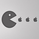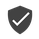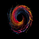4168 просмотров
4168
Wave Channel 3D
Built by Ricardo idea from JR & Aloakdutt from indieTrades Jan. 2010

This indicator is very easy to build. We utilize Moving Averages with a set multiplier and an offset. Specially we try to use Fibonacci sequence series numbers (1, 2, 3, 5, 8, 13, 21, 34, 55, 89, 144...) as time space and multiplier (default 89, 8). Also included is Donchian Channel to locate strong trends and possible future support - resistance.

Examples of support/resistance on chart.

Dominant Price Trends

Future Support Resistance

Comparing Fibonacci Series Time Space - Multiplier

When Comparing make note of confluence support/resistance showing up with Fibonacci Series
Example uses DC

When Comparing make note of confluence support/resistance showing up with Fibonacci Series
Example without DC / Smooth MA
study(title="3D-Wave Channel", shorttitle="3D-WC", overlay=true)
timespace = input(1)
smooth = input(89)
offsetMultiplier=input(8)
useDonchianAverage = input(false)
src = input(ohlc4)
ma = useDonchianAverage ? avg(highest(smooth),lowest(smooth)) : ema(src, smooth)
plot(ma, color=black, offset=offsetMultiplier*01)
plot(ma[timespace*01], color=silver, offset=offsetMultiplier*02)
plot(ma[timespace*02], color=silver, offset=offsetMultiplier*03)
plot(ma[timespace*03], color=gray, offset=offsetMultiplier*04)
plot(ma[timespace*04], color=gray, offset=offsetMultiplier*05)
plot(ma[timespace*05], color=gray, offset=offsetMultiplier*06)
plot(ma[timespace*06], color=silver, offset=offsetMultiplier*07)
plot(ma[timespace*07], color=silver, offset=offsetMultiplier*08)
plot(ma[timespace*08], color=gray, offset=offsetMultiplier*09)
plot(ma[timespace*09], color=gray, offset=offsetMultiplier*10)
plot(ma[timespace*10], color=black, offset=offsetMultiplier*11)

max_ma()=>max(ma[timespace*10],max(ma[timespace*9],max(ma[timespace*8],max(ma[timespace*7],max(ma[timespace*6],max(ma[timespace*5],max(ma[timespace*4],max(ma[timespace*3],max(ma[timespace*2],max(ma[timespace*1], ma))))))))))
min_ma()=>min(ma[timespace*10],min(ma[timespace*9],min(ma[timespace*8],min(ma[timespace*7],min(ma[timespace*6],min(ma[timespace*5],min(ma[timespace*4],min(ma[timespace*3],min(ma[timespace*2],min(ma[timespace*1], ma))))))))))

top = highest(max_ma(), smooth)
bot = lowest(min_ma(), smooth)

plot(top, color=black, offset=offsetMultiplier)
plot(bot, color=black, offset=offsetMultiplier)Today maybe it would have been more accurate to use this indicator, my stop loss support was broken too early
ОтветитьGreen live VS Grey line
I MISSED THE POP
ОтветитьWe can verify with my the S/R I previously draw. It's not far from reality, I recommend to use it as a reference and validate with a line previous S/R more precisely.
ОтветитьAn average is not a key level, depending which Time Frame you are using ... you will find the sweet spots in every Timeframes, but if you use it alone on one time frame, it will have some lags
Ответить